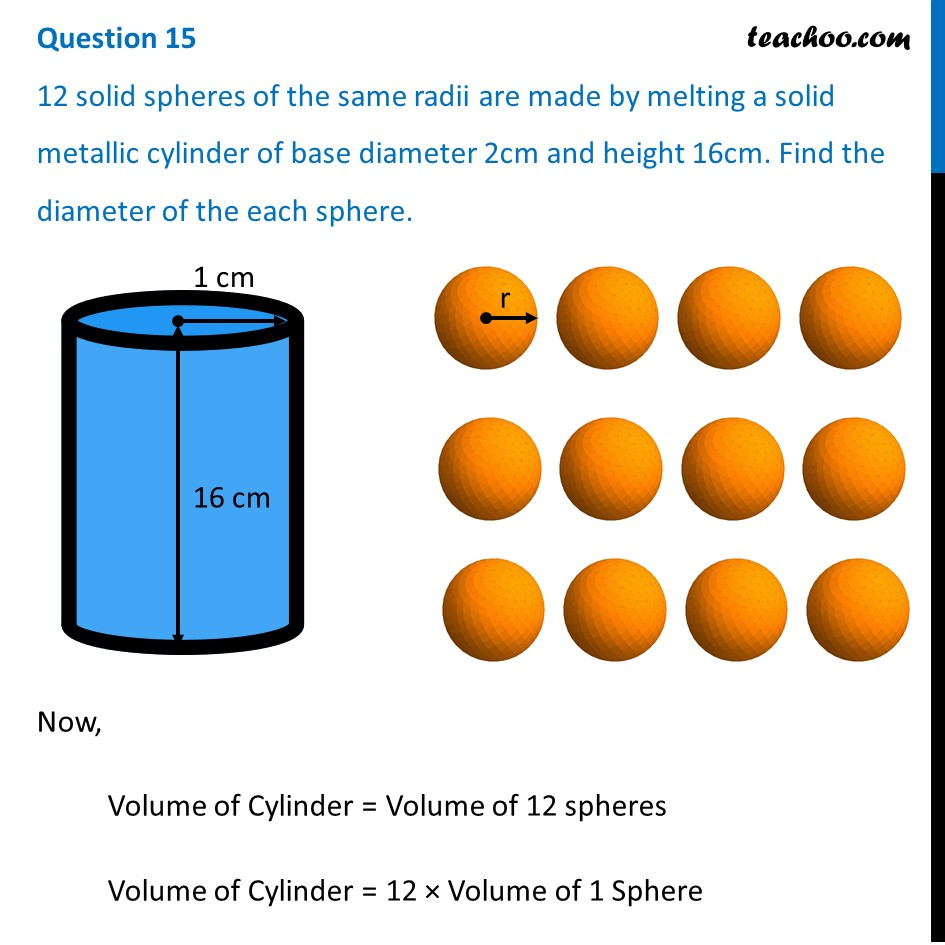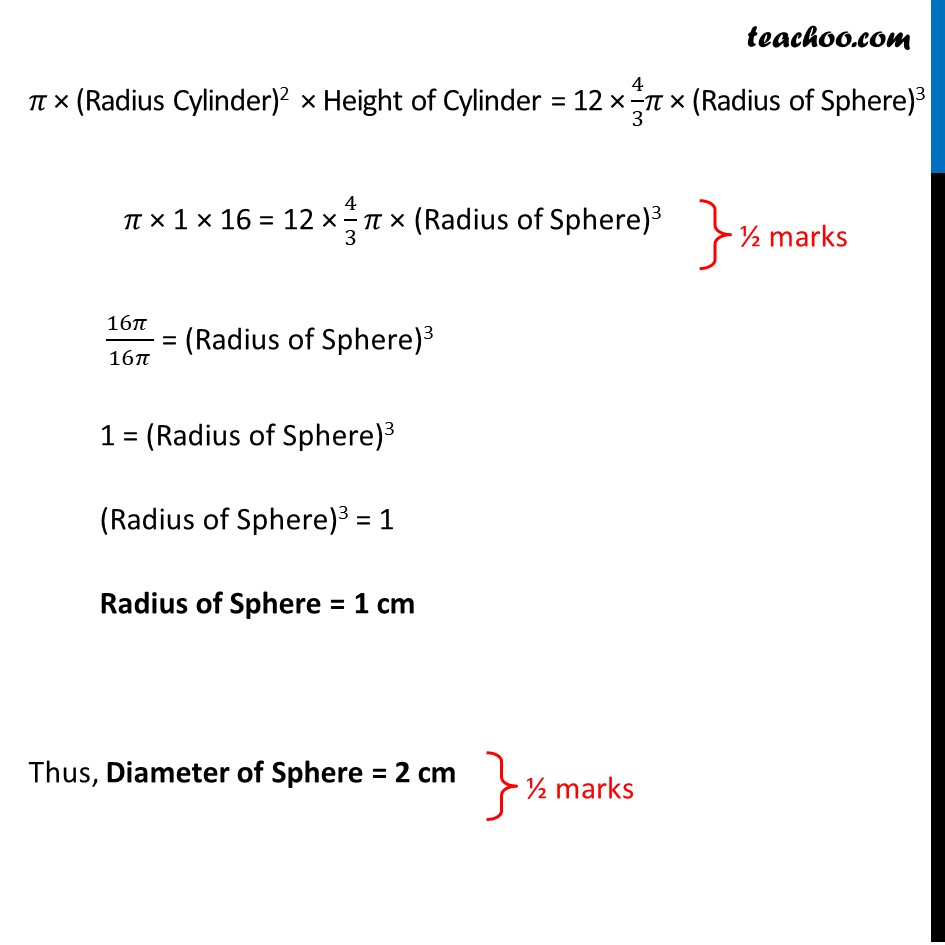12 solid spheres of the same radii are made by melting a solid metallic cylinder of base diameter 2cm and height 16cm. Find the diameter of the each sphere.1. Class 10
2. Solutions of Sample Papers for Class 10 Boards
3. CBSE Class 10 Sample Paper for 2021 Boards - Maths Standard

Transcript

Question 15 12 solid spheres of the same radii are made by melting a solid metallic cylinder of base diameter 2cm and height 16cm. Find the diameter of the each sphere. Now, Volume of Cylinder = Volume of 12 spheres Volume of Cylinder = 12 × Volume of 1 Sphere 𝜋 × (Radius Cylinder)2 × Height of Cylinder = 12 × 4/3𝜋 × (Radius of Sphere)3 𝜋 × 1 × 16 = 12 × 4/3 𝜋 × (Radius of Sphere)3 (16𝜋 )/16𝜋 = (Radius of Sphere)3 1 = (Radius of Sphere)3 (Radius of Sphere)3 = 1 Radius of Sphere = 1 cm Thus, Diameter of Sphere = 2 cm

CBSE Class 10 Sample Paper for 2021 Boards - Maths Standard

Class 10
Solutions of Sample Papers for Class 10 Boards